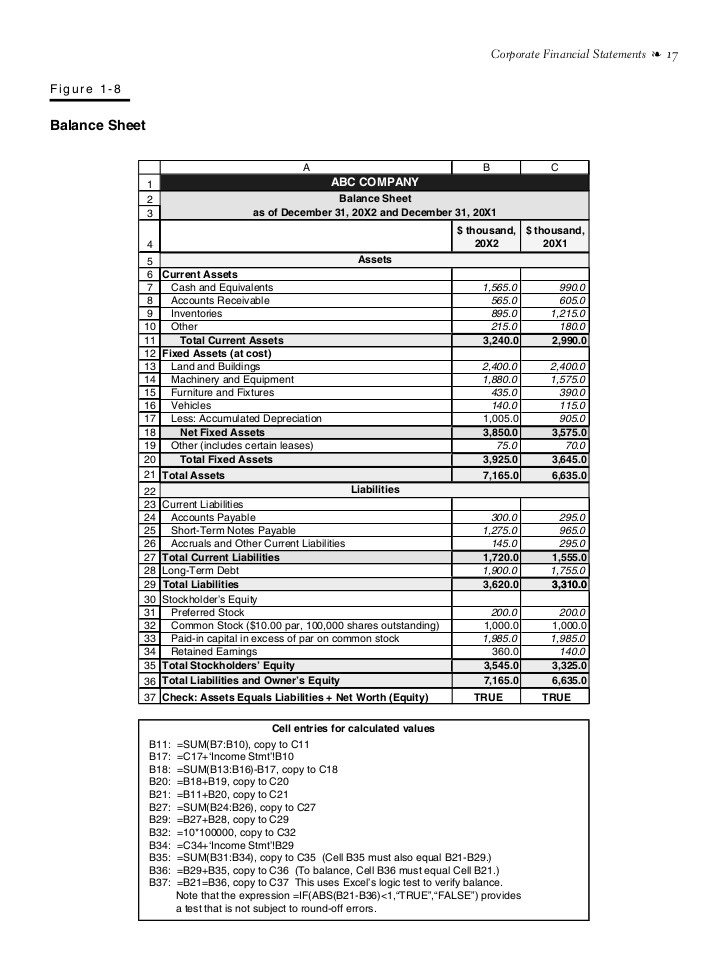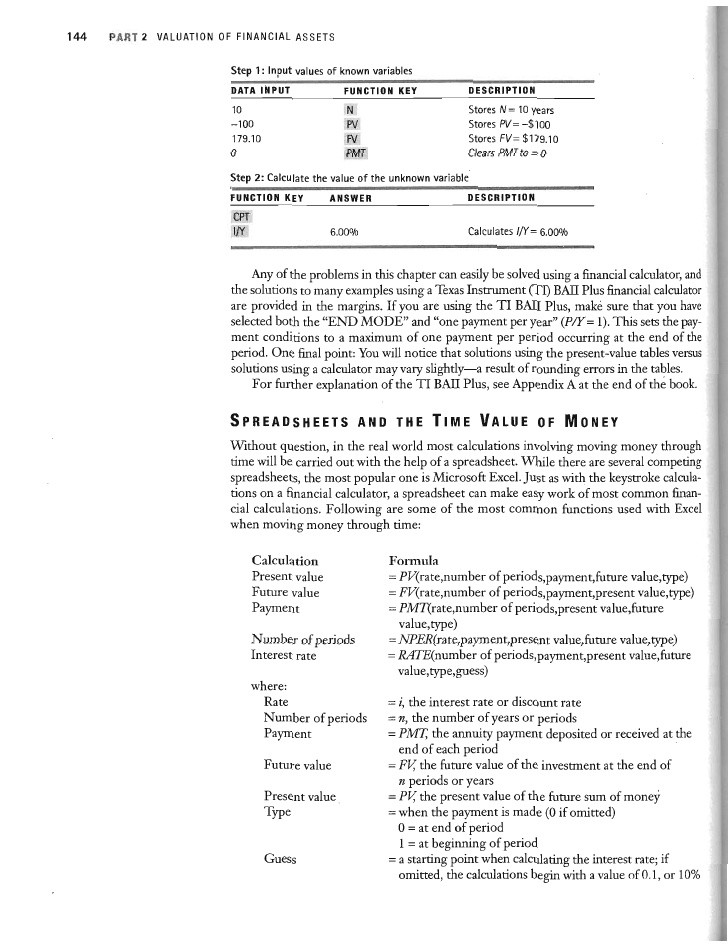Post on: 13 Октябрь, 2016“A dollar today is worth more than a dollar tomorrow.” You may have heard this phrase few times before and there is a reason for that: time value of money needs to be considered in every financial analysis that covers more than one time period. This is also the first basic principle of finance. So, it may be useful to explain what this means.

Since it’s usually easier to explain concepts using examples, suppose you can invest \$100 today to get \$120 a year from now. To make the right investment choice, you would need to know what is today’s value of these future \$120 and then compare it with today’s investment of \$100. Today’s value of future cash flow is called the present value. The present value in our example must be less than \$120 since today you could invest the money to start earning interest immediately.

The present value of a delayed cash flow can be found by multiplying the cash flow by a discount factor. Mathematically speaking, the discount factor is expressed as the reciprocal of 1 plus a rate of return as shown in the formula below. (The formula below would need to be adjusted if there is more than one time period being evaluated, but this is being omitted here for simplicity.)

The rate of return r is the reward that investors demand for accepting delayed payments. The rate describes the return offered by comparable investment alternatives. This rate of return is also referred to as the discount rate. hurdle rate. or opportunity cost of capital .

Coming back to our example, we need to discount expected future cash flows to arrive at their present value. Let’s assume for now that comparable investment alternatives yield 6 percent interest. Hence, our rate of return would be 6 percent and the present value of the \$120 a year from now would be \$113 as shown below.Now, let’s compare this present value with today’s investment of \$100. We can calculate the net present value (NPV) of \$13 by subtracting today’s investment from the present value as shown below. Since NPV is positive, it would make financial sense to make this investment, everything else being equal.

The time value of money concept described here helps us to calculate the present value of a cash flow in a future time period, so we can express future cash flows in today’s dollars (or any other currency). And with NPV we can compare cash flows covering more than one time period.

More from this section
Categories
Tags

• Most Popular